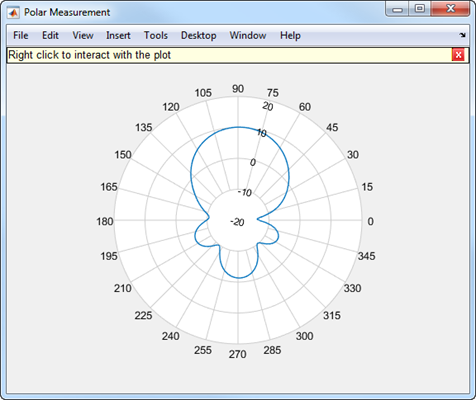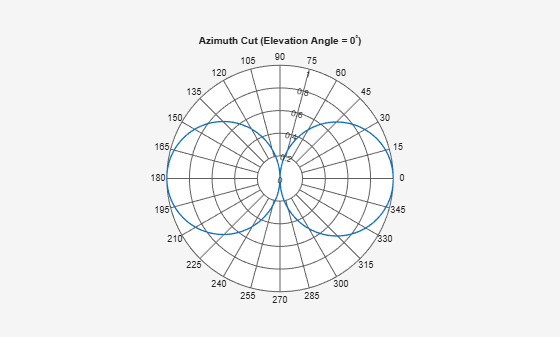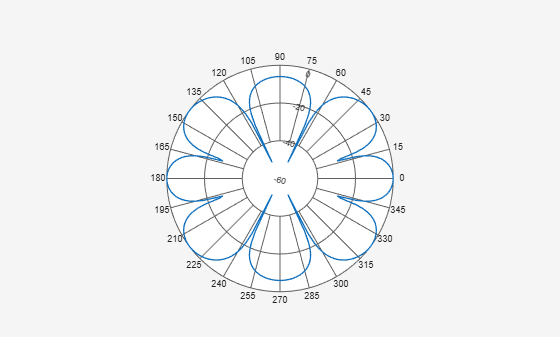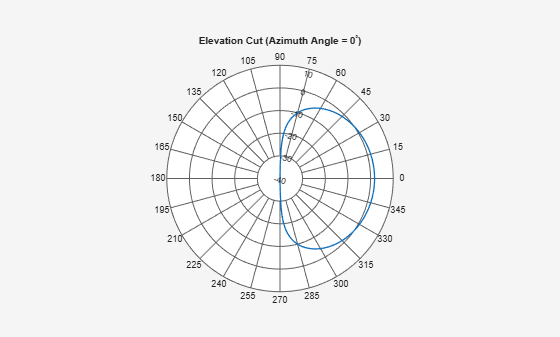# polarpattern class

Interactive plot of radiation patterns in polar format

## Description`polarpattern` class plots antenna or array radiation patterns in interactive polar format. You can also plot other types of polar data. Use these plots when interactive data visualization or measurement is required. Right-click the Polar Measurement window to change the properties, zoom in, or add more data to the plot.

## Construction

````polarpattern` plots antenna or array radiation patterns and other types of data in polar format. `polarpattern` plots field value data of radiation patterns for visualization and measurement. Right-click the polar plot to interact.```

example

````polarpattern(data)` creates a polar plot with magnitude values in the vector `d`. In this polar plot, angles are uniformly spaced on the unit circle, starting at `0` degrees.```

example

````polarpattern(angle,magnitude)` creates a polar plot from a set of angle vectors and corresponding magnitudes. You can also create polar plots from multiple sets for angle vectors and corresponding sets of magnitude using the syntax: ```polarpattern(angle1, magnitude1, angle2, magnitude2...)```.```
````p = polarpattern(___)` returns an object handle that you can use to customize the plot or add measurements. You can specify any of the arguments from the previous syntaxes.```
````p = polarpattern('gco')` returns an object handle from polar pattern in the current figure.```

example

````polarpattern(___,Name,Value)` creates a polar plot, with additional properties specified by one or more name-value pair arguments. `Name` is the property name and `Value` is the corresponding property value. You can specify several name-value pair arguments in any order as `Name1`, `Value1`, `...`, `NameN`, `ValueN`. Properties not specified retain their default values. To list all the property `Name,Value` pairs, use `details(p)`. To list all the property `Name,Value` pairs, use `details(p)`. You can use the properties to extract any data from the radiation pattern from the polar plot. For example, ```p = polarpattern(data,'Peaks',3)``` identifies and displays the three highest peaks in the pattern data. For a list of properties, see PolarPattern Properties.```
````polarpattern(ax,___)` creates a polar plot using axes handle, `ax` instead of the current axes handle.```

## Input Arguments

expand all

Antenna or array data, specified as one of the following:

• A real length-M vector, where M contains the magnitude values with angles assumed to be $\frac{\left(0:M-1\right)}{M}×{360}^{\circ }$ degrees.

• A real M-by-N matrix, where M contains the magnitude values and N contains the independent data sets. Each column in the matrix has angles taken from the vector $\frac{\left(0:M-1\right)}{M}×{360}^{\circ }$ degrees.

• A real N-D array, where N is the number of dimensions. Arrays with dimensions `2` and greater are independent data sets.

• A complex vector or matrix, where `data` contains Cartesian coordinates (x, y) of each point. x contains the real (`data`) and y contains the imaginary (`data`).

When data is in a logarithmic form, such as dB, magnitude values can be negative. In this case,`polarpattern` plots the smallest magnitude values at the origin of the polar plot and largest magnitude values at the maximum radius.

Set of angles, specified as a vector in degrees.

Set of magnitude values, specified as a vector or a matrix. For a matrix of magnitude values, each column is an independent set of magnitude values and corresponds to the same set of angles.

## Methods

 `add` Add data to existing polar plot `addCursor` Add cursor to polar plot angle `animate` Replace existing data with new data for animation `createLabels` Create legend labels `findLobes` Main, back and side lobe data `replace` Replace existing data with new data in polar plot `showPeaksTable` Show or hide peak marker table `showSpan` Show or hide angle span between two markers

## Examples

collapse all

Specify a cosine antenna pattern from 0° to 360° in azimuth at 0° elevation. Then, plot the antenna pattern using `polarpattern`.

Create the pattern.

```az = [0:360]; p = abs(cosd(az));```

Plot the polar pattern of the antenna for an azimuth cut at 0° elevation.

`polarpattern(p,'TitleTopTextInterpreter','tex','TitleTop','Azimuth Cut (Elevation Angle = 0^{\circ})');`Construct a 3-by-2 rectangular lattice URA. By default, the array consists of isotropic antenna elements. Assume the operating frequency is 1 GHz. Then, plot the antenna pattern using `polarpattern`.

Create the array.

```array = phased.URA('Size',[3 2]); fc = 1.0e9;```

Plot the polar pattern of the array for an elevation cut at 0° azimuth.

```c = physconst('LightSpeed'); p = pattern(array,fc,[-180:180],0,'PropagationSpeed',c,'CoordinateSystem',... 'polar','Type','powerdb','Normalize',true); polarpattern([-180:180],p);```Specify a short-dipole antenna with the dipole oriented along the z-axis and operating at 250 MHz. Then, plot the antenna pattern using `polarpattern` and specifying a title.

Create the short-dipole antenna element System object™.

```antenna = phased.ShortDipoleAntennaElement('FrequencyRange',[100e6,600e6],... 'AxisDirection','Z'); fc = 250.0e6;```

Plot the polar pattern of the antenna for an elevation cut at 0° azimuth.

```v = pattern(antenna,fc,0,-90:90); polarpattern([-90:90],v,'TitleTopTextInterpreter','tex',... 'TitleTop','Elevation Cut (Azimuth Angle = 0^{\circ})');```Specify a short-dipole antenna with the dipole oriented along the z-axis and operating at 250 MHz. Then, plot the antenna pattern using `polarpattern` and specifying a title.

Create the short-dipole antenna element System object™.

```antenna = phased.ShortDipoleAntennaElement('FrequencyRange',[100e6,600e6],... 'AxisDirection','Z'); fc = 250.0e6;```

Create the polar pattern of the antenna for an elevation cut at 0° azimuth.

```p = pattern(antenna,fc,0,-90:90); P = polarpattern([-90:90],p,'TitleTopTextInterpreter','tex',... 'TitleTop','Elevation Cut (Azimuth Angle = 0^{\circ})');```Display the properties of the plot.

`details(P)`
``` internal.polari handle with properties: Interactive: 1 LegendLabels: '' AntennaMetrics: 0 CleanData: 0 AngleData: [181x1 double] MagnitudeData: [181x1 double] IntensityData: [] AngleMarkers: [0x1 struct] CursorMarkers: [0x1 struct] PeakMarkers: [0x1 struct] ActiveDataset: 1 AngleLimVisible: 0 LegendVisible: 0 Span: 0 TitleTop: 'Elevation Cut (Azimuth Angle = 0^{\circ})' TitleBottom: '' Peaks: [] FontSize: 10 MagnitudeLim: [-40 10] MagnitudeAxisAngle: 75 MagnitudeTick: [-40 -30 -20 -10 0 10] MagnitudeTickLabelColor: 'k' AngleLim: [0 360] AngleTickLabel: {1x24 cell} AngleTickLabelColor: 'k' TitleTopFontSizeMultiplier: 1.1000 TitleBottomFontSizeMultiplier: 0.9000 TitleTopFontWeight: 'bold' TitleBottomFontWeight: 'normal' TitleTopTextInterpreter: 'tex' TitleBottomTextInterpreter: 'none' TitleTopOffset: 0.1500 TitleBottomOffset: 0.1500 ToolTips: 1 MagnitudeLimBounds: [-Inf Inf] MagnitudeFontSizeMultiplier: 0.9000 AngleFontSizeMultiplier: 1 AngleAtTop: 90 AngleDirection: 'ccw' AngleResolution: 15 AngleTickLabelRotation: 0 AngleTickLabelFormat: '360' AngleTickLabelColorMode: 'contrast' PeaksOptions: {} AngleTickLabelVisible: 1 Style: 'line' DataUnits: 'dB' DisplayUnits: 'dB' NormalizeData: 0 ConnectEndpoints: 0 DisconnectAngleGaps: 0 EdgeColor: 'k' LineStyle: '-' LineWidth: 1 FontName: 'Helvetica' FontSizeMode: 'auto' GridForegroundColor: [0.8000 0.8000 0.8000] GridBackgroundColor: 'w' DrawGridToOrigin: 0 GridOverData: 0 GridAutoRefinement: 0 GridWidth: 0.5000 GridVisible: 1 ClipData: 1 TemporaryCursor: 1 MagnitudeLimMode: 'auto' MagnitudeAxisAngleMode: 'auto' MagnitudeTickMode: 'auto' MagnitudeTickLabelColorMode: 'contrast' MagnitudeTickLabelVisible: 1 MagnitudeUnits: '' IntensityUnits: '' Marker: 'none' MarkerSize: 6 Parent: [1x1 Figure] NextPlot: 'replace' ColorOrder: [7x3 double] ColorOrderIndex: 1 SectorsColor: [16x3 double] SectorsAlpha: 0.5000 View: 'full' ZeroAngleLine: 0 ```